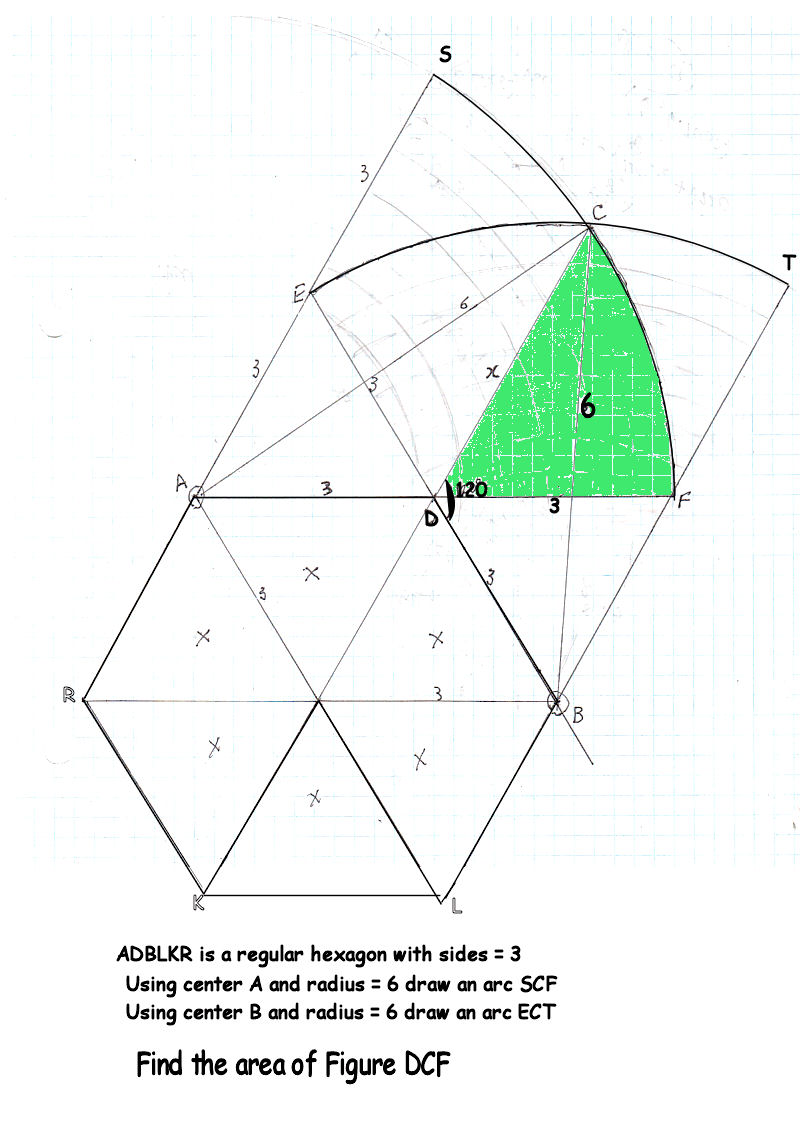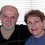# Finding AreaI posted a problem "How Much Can a Goat Eat #2". Using a false assumption I calculated the overlapped area incorrectly. I request assistance in this regard. Please calculate the area of the green colored figure in this image. Thanks.Note by Guiseppi Butel
6 years, 8 months ago

This discussion board is a place to discuss our Daily Challenges and the math and science related to those challenges. Explanations are more than just a solution — they should explain the steps and thinking strategies that you used to obtain the solution. Comments should further the discussion of math and science.

When posting on Brilliant:

• Use the emojis to react to an explanation, whether you're congratulating a job well done , or just really confused .
• Ask specific questions about the challenge or the steps in somebody's explanation. Well-posed questions can add a lot to the discussion, but posting "I don't understand!" doesn't help anyone.
• Try to contribute something new to the discussion, whether it is an extension, generalization or other idea related to the challenge.
• Stay on topic — we're all here to learn more about math and science, not to hear about your favorite get-rich-quick scheme or current world events.

MarkdownAppears as
*italics* or _italics_ italics
**bold** or __bold__ bold
- bulleted- list
• bulleted
• list
1. numbered2. list
1. numbered
2. list
Note: you must add a full line of space before and after lists for them to show up correctly
paragraph 1paragraph 2

paragraph 1

paragraph 2

[example link](https://brilliant.org)example link
> This is a quote
This is a quote
    # I indented these lines
# 4 spaces, and now they show
# up as a code block.

print "hello world"
# I indented these lines
# 4 spaces, and now they show
# up as a code block.

print "hello world"
MathAppears as
Remember to wrap math in $$ ... $$ or $ ... $ to ensure proper formatting.
2 \times 3 $2 \times 3$
2^{34} $2^{34}$
a_{i-1} $a_{i-1}$
\frac{2}{3} $\frac{2}{3}$
\sqrt{2} $\sqrt{2}$
\sum_{i=1}^3 $\sum_{i=1}^3$
\sin \theta $\sin \theta$
\boxed{123} $\boxed{123}$

## Comments

Sort by:

Top Newest

It looks like there are several ways to go about this, but this is my initial thought. First, we have $AB = 3\sqrt{3}$. Thus $\theta = \angle CAD = \cos^{-1}(\frac{\sqrt{3}}{4}) - \frac{\pi}{6}$. The area of the green region will then be the area of sector $CAF$ minus the area of $\Delta CAD$. This comes out to

$(\frac{1}{2})(6^{2})\theta - (\frac{1}{2})(3)(6\sin(\theta)) = 18\theta - 9\sin(\theta)$.

This "simplifies" to

$18\cos^{-1}(\frac{\sqrt{3}}{4}) - 3\pi - (\frac{9}{8})\sqrt{3}(\sqrt{13} - 1)$,

which equals $5.7115$ to $4$ decimal places.

- 6 years, 8 months ago

Log in to reply

Thanks, Brian. I obtained the same answer by using a different method. In the sector ACF I calculated A by Law of Sines to be 34.34109373 degrees, altitude from AC to D to be 1.692355197.

Area of the green = Area of the sector - Area of triangle ADC

Area of the green = (34.34.../360 * Pi * 6^2) - (1/2 * 6* 1.69..)

Area of the green = 10.788573 - 5.0770656 = 5.7115074

This amount was taken to the problem "How Much Can a Goat Eat #2" and a corrected answer was obtained.

- 6 years, 8 months ago

Log in to reply

Glad we came to the same conclusion. I enjoyed the goat problem; thanks for posting it. :)

- 6 years, 8 months ago

Log in to reply

yup its a good sum

- 6 years, 8 months ago

Log in to reply

wow

- 6 years, 7 months ago

Log in to reply

5.711

- 6 years, 7 months ago

Log in to reply

Yup got 5.711

- 6 years, 7 months ago

Log in to reply

49

- 6 years, 7 months ago

Log in to reply

4.92 square units

- 6 years, 7 months ago

Log in to reply

The area is 8.58

- 6 years, 7 months ago

Log in to reply

How did you get that answer?

- 6 years, 7 months ago

Log in to reply

it can be solved by using integration in a very easy manner .just shift the whole diagram on a xy plane and do the integration by writing the equation .

- 6 years, 7 months ago

Log in to reply

5.748

- 6 years, 7 months ago

Log in to reply

5.7115 Where did you err?

- 6 years, 7 months ago

Log in to reply

what is the correct answer of this problem ????

- 6 years, 6 months ago

Log in to reply

Look back at what Brian and I posted on Oct. 20.

If you think that you have the correct answer please submit your calculations.

- 6 years, 6 months ago

Log in to reply

as i tried to solve it i got 4.9 as area of shaded region

- 6 years, 6 months ago

Log in to reply

×

Problem Loading...

Note Loading...

Set Loading...